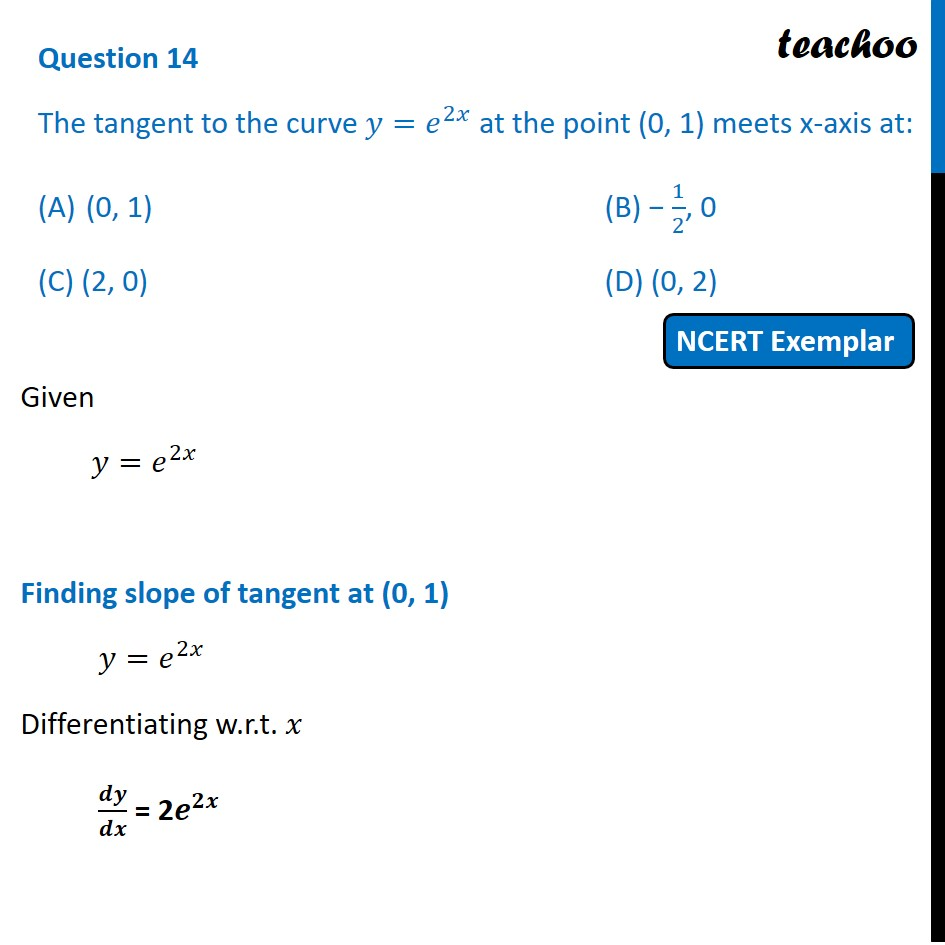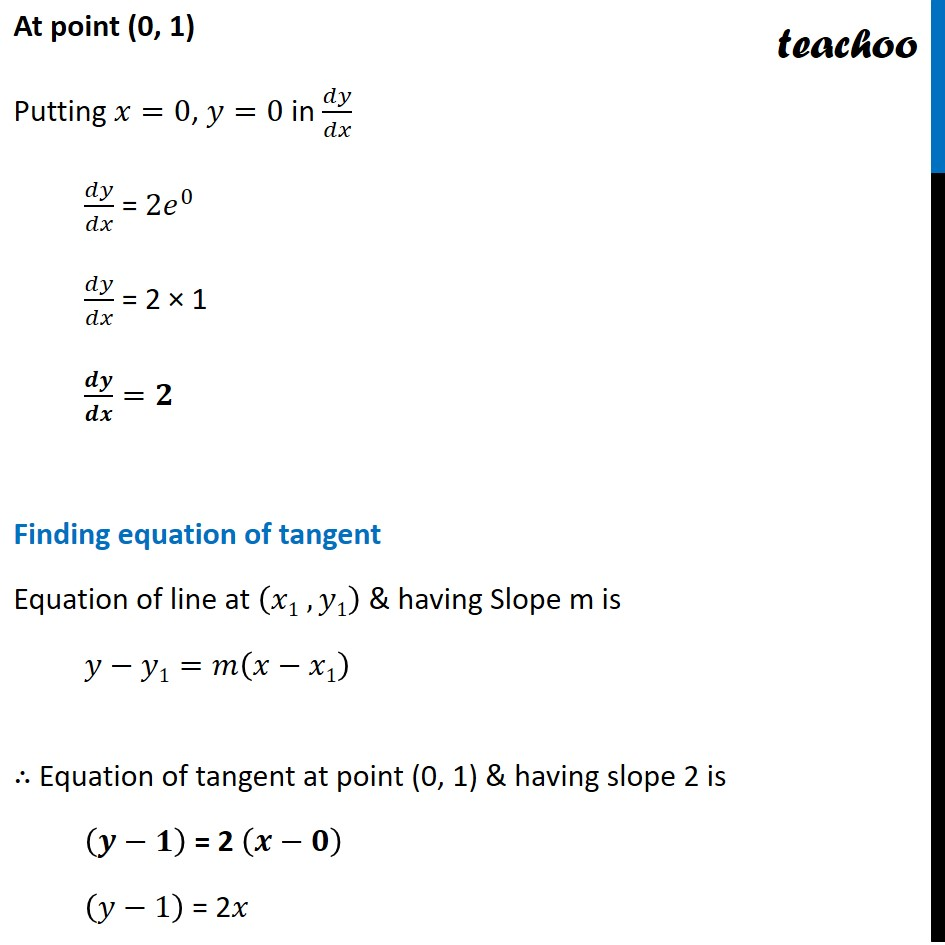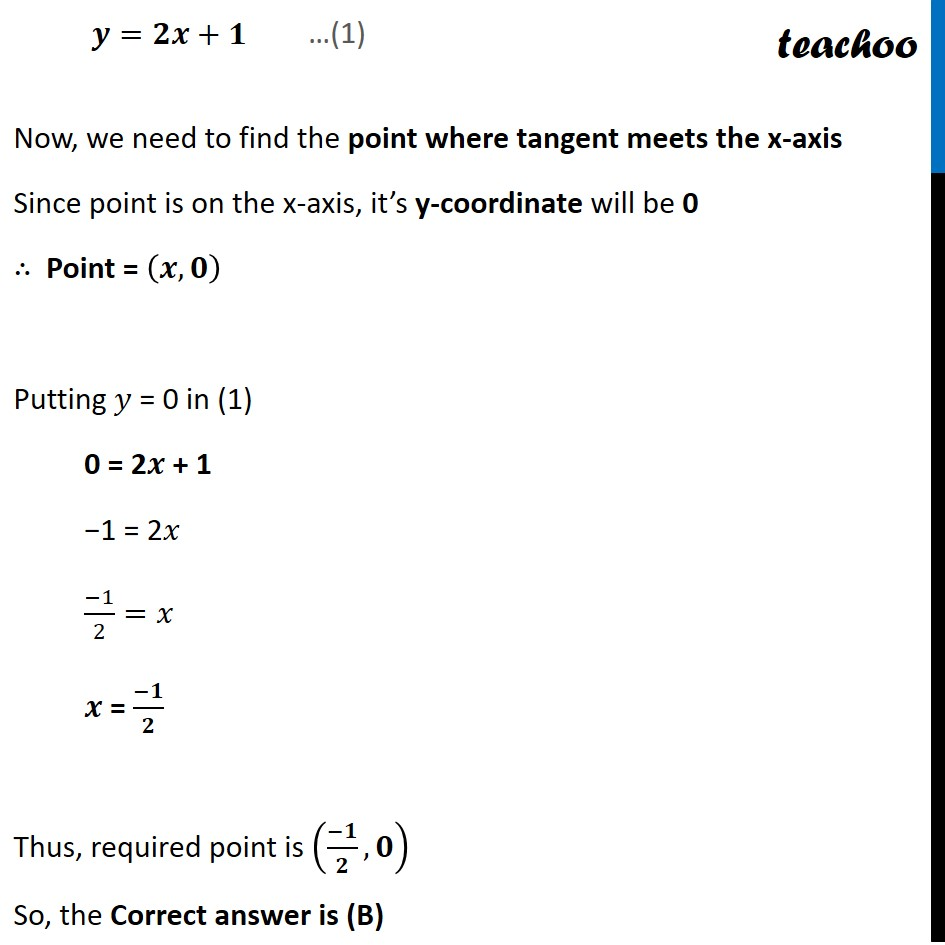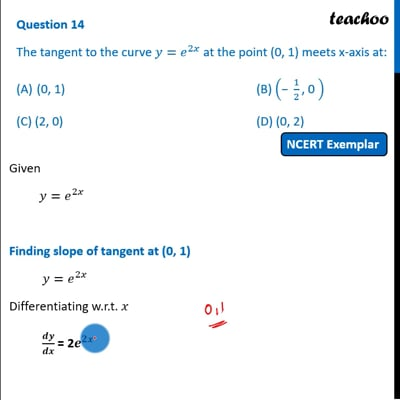NCERT Exemplar - MCQs

Chapter 6 Class 12 Application of Derivatives
Serial order wise

## (C) (2, 0)         (D) (0, 2)This video is only available for Teachoo black users

Learn in your speed, with individual attention - Teachoo Maths 1-on-1 Class

### Transcript

Question 12 The tangent to the curve 〖𝑦=𝑒〗^2𝑥 at the point (0, 1) meets x-axis at: (0, 1) (B) − 1/2, 0 (C) (2, 0) (D) (0, 2) Given 〖𝑦=𝑒〗^2𝑥 Finding slope of tangent at (0, 1) 〖𝑦=𝑒〗^2𝑥 Differentiating w.r.t. 𝑥 𝒅𝒚/𝒅𝒙 = 2𝒆^𝟐𝒙 At point (0, 1) Putting 𝑥=0, 𝑦=0 in 𝑑𝑦/𝑑𝑥 𝑑𝑦/𝑑𝑥 = 〖2𝑒〗^0 𝑑𝑦/𝑑𝑥 = 2 × 1 𝒅𝒚/𝒅𝒙=𝟐 Finding equation of tangent Equation of line at (𝑥1 , 𝑦1) & having Slope m is 𝑦−𝑦1=𝑚(𝑥−𝑥1) ∴ Equation of tangent at point (0, 1) & having slope 2 is (𝒚−𝟏) = 2 (𝒙−𝟎) (𝑦−1) = 2𝑥 𝒚=𝟐𝒙+𝟏 Now, we need to find the point where tangent meets the x-axis Since point is on the x-axis, it’s y-coordinate will be 0 ∴ Point = (𝒙,𝟎) Putting 𝑦 = 0 in (1) 0 = 2𝒙 + 1 −1 = 2𝑥 (−1)/2=𝑥 𝒙 = (−𝟏)/𝟐 Thus, required point is ((−𝟏)/𝟐, 𝟎) So, the Correct answer is (B)